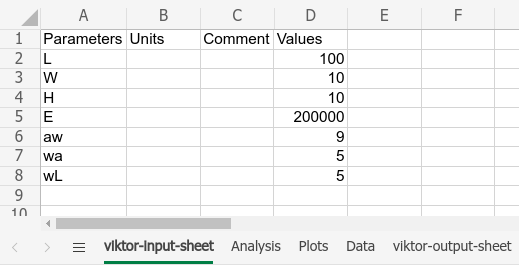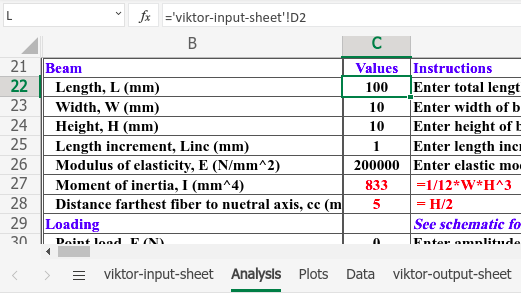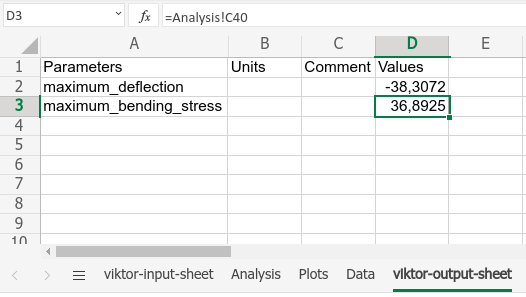# Using a calculation spreadsheet

In this tutorial we will create an application to analyze a simply supported beam under load. A spreadsheet will be used for the calculations.

• The starting app for this tutorial can be downloaded here.
• The end result can be downloaded here.

Download the starting app to your hard disk. Run the `install` command from within the app folder to install the app and its dependencies, `clear` any previous saved local database, and run `start` to start to app:

``viktor-cli installviktor-cli clearviktor-cli start``

In the browser, click the "Example" entity to open its editor and verify the app is installed and running as expected.

## Folder structure​

The app has the following folder structure:

``spreadsheet-tutorial├── app.py├── beam_calculation.xls ├── beam_schematic.svg├── requirements.txt└── viktor.config.toml``

 source: https://stuff.mit.edu (04-05-2020)

Open `app.py` and have a look at the code that we have set up for you. Note that the code already has some basic class structure. We will extend the code, step-by-step, throughout this tutorial.

## Preparing the spreadsheet​

To be able to use a spreadsheet as a calculation tool within a VIKTOR app, a few modifications must be performed to the spreadsheet file. Open `beam_calculation.xls` and add two sheets: `viktor-input-sheet` and `viktor-output-sheet`. These sheets contain the input and output values respectively. In the `viktor-input-sheet`, add the following data:The values from `viktor-input-sheet` should now be used in the `Analysis` sheet. For example, cell C22 below refers to the "L" parameter defined in the `viktor-input-sheet` sheet:In the `viktor-output-sheet` the desired output parameters are defined, with their values taken from the `Analysis` sheet:You should now be able to change the values on the `viktor-input-sheet`, resulting in changed values in the `viktor-output-sheet`.

## Coupling the spreadsheet​

The spreadsheet is now ready for use within the app. VIKTOR provides a helper class specifically for this task: `SpreadsheetCalculation`. Open `app.py` and replace the method `get_data_view` with the following code (mind the extra imports):

``from viktor.external.spreadsheet import SpreadsheetCalculationInput, SpreadsheetCalculationclass Calculation(ViktorController):    ...    @DataView('Results', duration_guess=1)    def get_data_view(self, params, **kwargs):        inputs = [            SpreadsheetCalculationInput('L', params.beam.length),            SpreadsheetCalculationInput('W', params.beam.width),            SpreadsheetCalculationInput('H', params.beam.height),            SpreadsheetCalculationInput('E', params.beam.E),            SpreadsheetCalculationInput('aw', params.loads.aw),            SpreadsheetCalculationInput('wa', params.loads.wa),            SpreadsheetCalculationInput('wL', params.loads.wL),        ]        sheet_path = Path(__file__).parent / 'beam_calculation.xls'        sheet = SpreadsheetCalculation.from_path(sheet_path, inputs=inputs)        result = sheet.evaluate(include_filled_file=True)        print(result.values)        data = DataGroup(            maximum_deflection=DataItem('Maximum deflection', -10, suffix='microns', number_of_decimals=2),            maximum_bending_stress=DataItem('Maximum bending stress', -13, suffix='N/mm2', number_of_decimals=2),        )        return DataResult(data)``

The input parameters are accessed from the params dictionary and follow the same structure as defined in the parametrization class.

In your browser change the value of `Length` from `100` to `120` and notice that the updated values are now printed to the command-line.

## Showing the output​

To display the output in the app's interface, the parameters from the `result` dictionary must be linked to the `DataItem`s, as shown below. Modify the method `get_data_view` as follows:

``    def get_data_view(self, params, **kwargs):        ...        data = DataGroup(            maximum_deflection=DataItem('Maximum deflection', result.values['maximum_deflection'], suffix='microns', number_of_decimals=2),            maximum_bending_stress=DataItem('Maximum bending stress', result.values['maximum_bending_stress'], suffix='N/mm2', number_of_decimals=2),        )        ...``

Confirm that changing the value in your browser gives you different outputs in the `DataView`.

A nice addition to the app would be that the sheet, used for the calculation, can be downloaded. Add a `DownloadButton` to the parametrization (mind the extra import):

``from viktor.parametrization import ..., DownloadButtonclass Parametrization(ViktorParametrization):    ...    downloads = Section('Downloads')    downloads.spreadsheet = DownloadButton('Download', 'download_spreadsheet')``

The `download_spreadsheet` method should contain the downloading logic and must be added in the `Calculation` class. To prevent writing duplicate code, however, we will first restructure this code a bit. Let's create a new method called `get_evaluated_spreadsheet`, which will be called by both `get_data_view` and `download_spreadsheet`:

``class Calculation(ViktorController):    ...    def get_evaluated_spreadsheet(self, params):        inputs = [            SpreadsheetCalculationInput('L', params.beam.length),            SpreadsheetCalculationInput('W', params.beam.width),            SpreadsheetCalculationInput('H', params.beam.height),            SpreadsheetCalculationInput('E', params.beam.E),            SpreadsheetCalculationInput('aw', params.loads.aw),            SpreadsheetCalculationInput('wa', params.loads.wa),            SpreadsheetCalculationInput('wL', params.loads.wL),        ]        sheet_path = Path(__file__).parent / 'beam_calculation.xls'        sheet = SpreadsheetCalculation.from_path(sheet_path, inputs=inputs)        result = sheet.evaluate(include_filled_file=True)        return result    @DataView('Results', duration_guess=1)    def get_data_view(self, params, **kwargs):        result = self.get_evaluated_spreadsheet(params)        max_deflection = result.values['maximum_deflection']        max_bending_stress = result.values['maximum_bending_stress']        data = DataGroup(            maximum_deflection=DataItem('Maximum deflection', max_deflection, suffix='microns', number_of_decimals=2),            maximum_bending_stress=DataItem('Maximum bending stress', max_bending_stress, suffix='N/mm2', number_of_decimals=2),        )        return DataResult(data)``

Now we add the method `download_spreadsheet`, which uses this newly created method (mind the extra import):

``...from viktor.result import DownloadResultclass Calculation(ViktorController):    ...    def download_spreadsheet(self, params, **kwargs):        result = self.get_evaluated_spreadsheet(params)        return DownloadResult(result.file_content, 'evaluated_beam.xlsx')``

Confirm that the download button functions as expected.